150724 Epaper

 A Section B SectionC Section D Section150723 Epaper

 A Section B SectionC Section D Section150722 Epaper

 A Section B SectionC Section D Section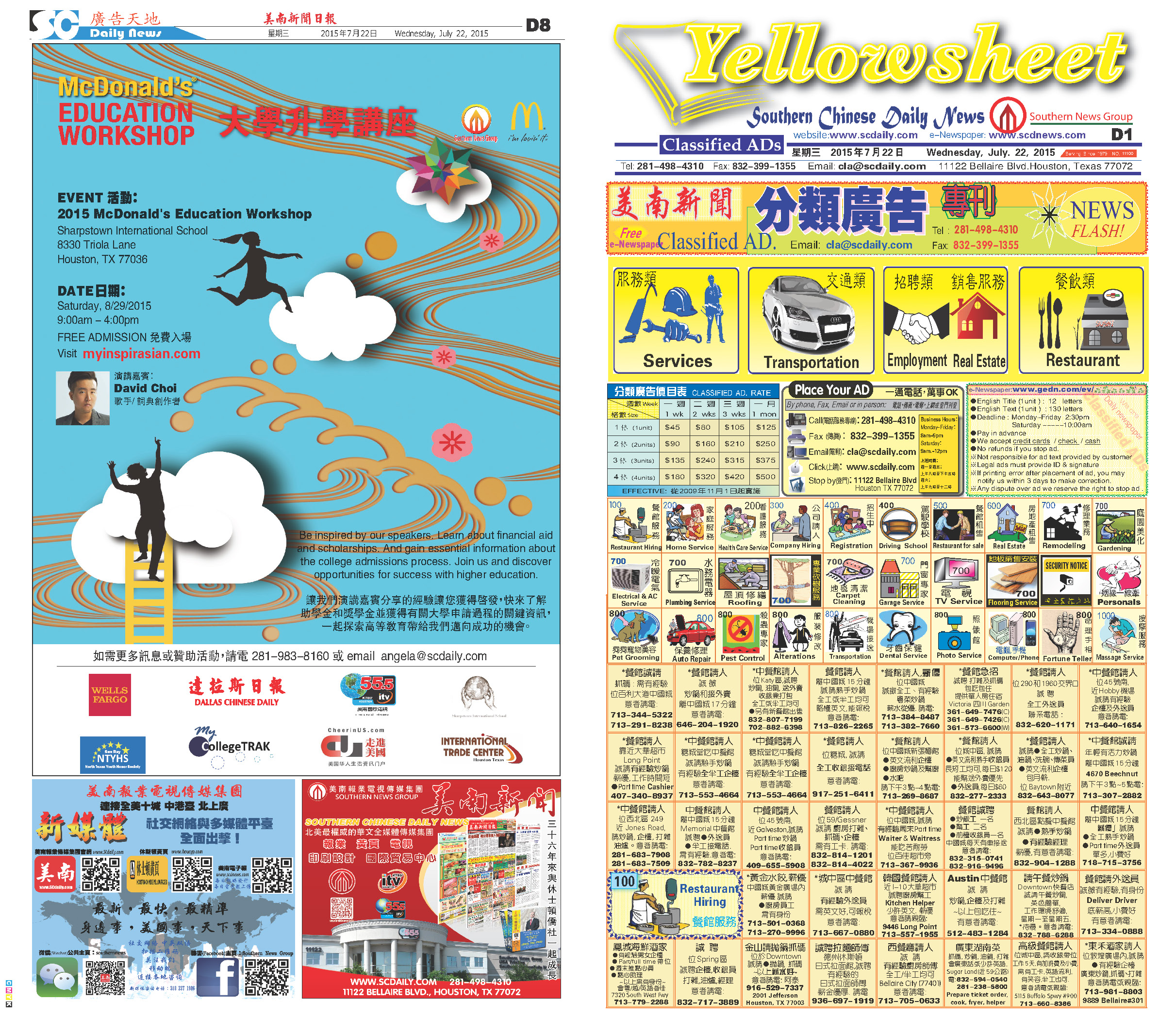150721 Epaper

 A Section B SectionC Section D Section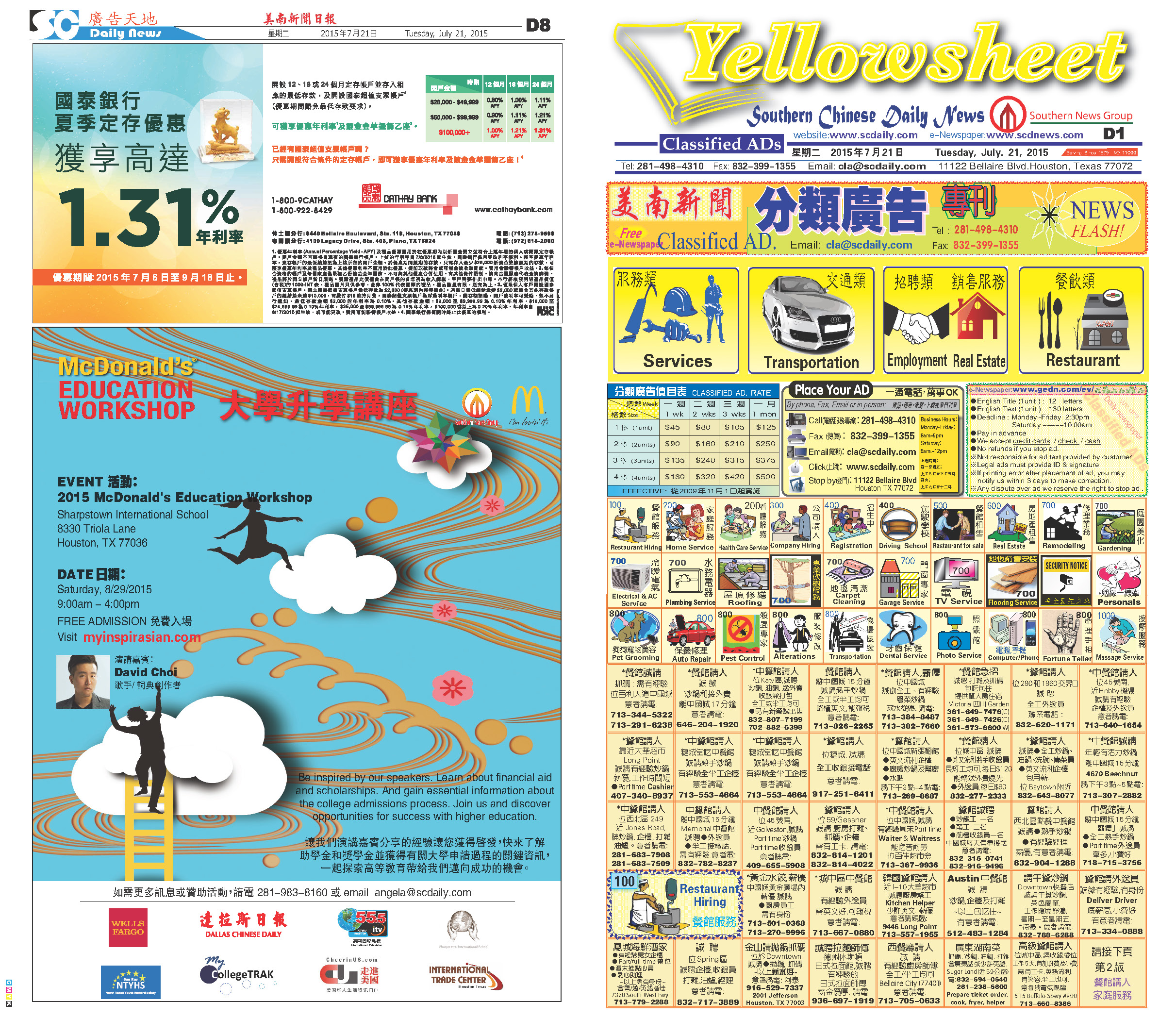150720 Epaper

 A Section B SectionC Section D Section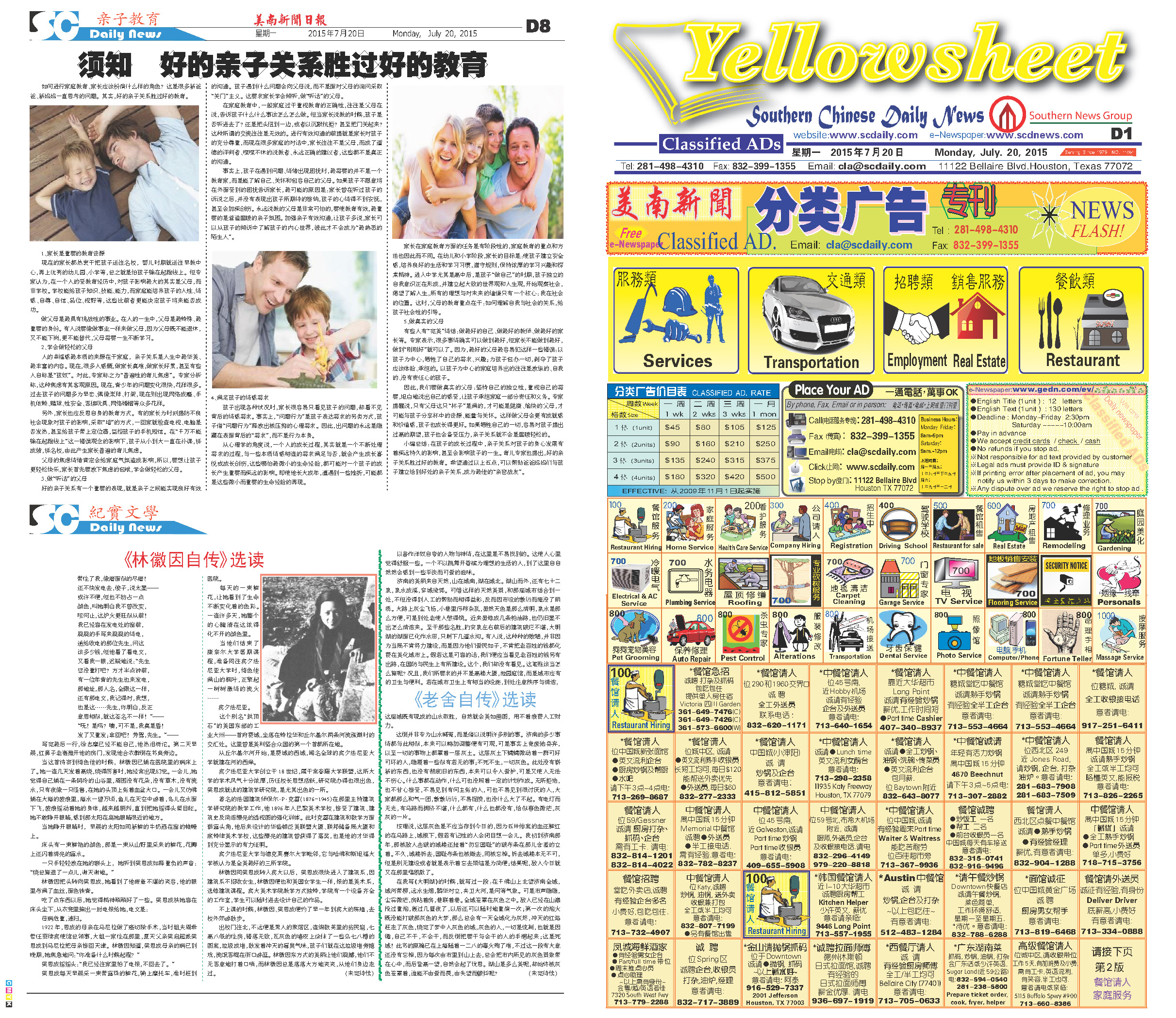150719 Epaper

 A Section B SectionC Section D Section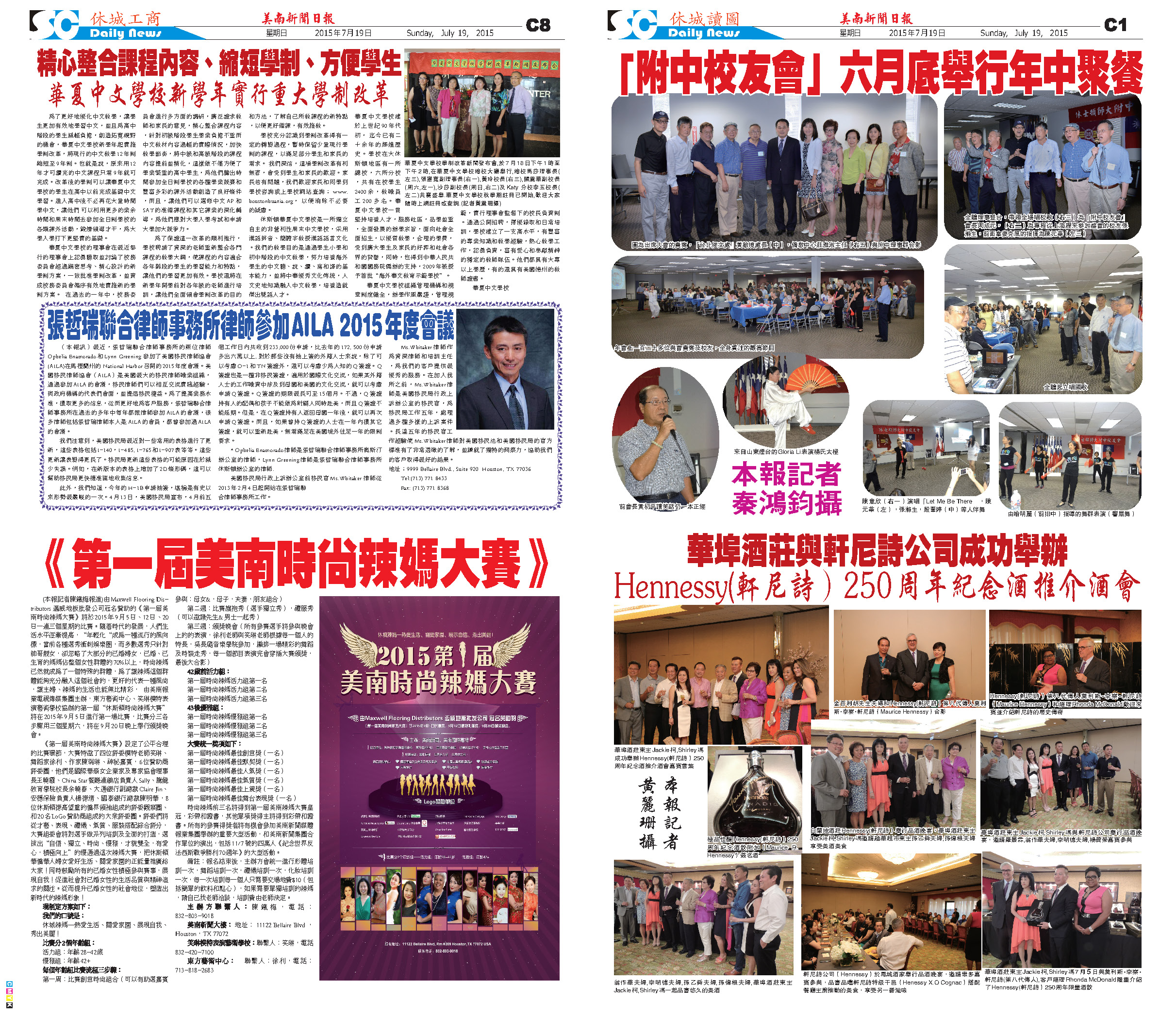150718 Epaper

 A Section B Section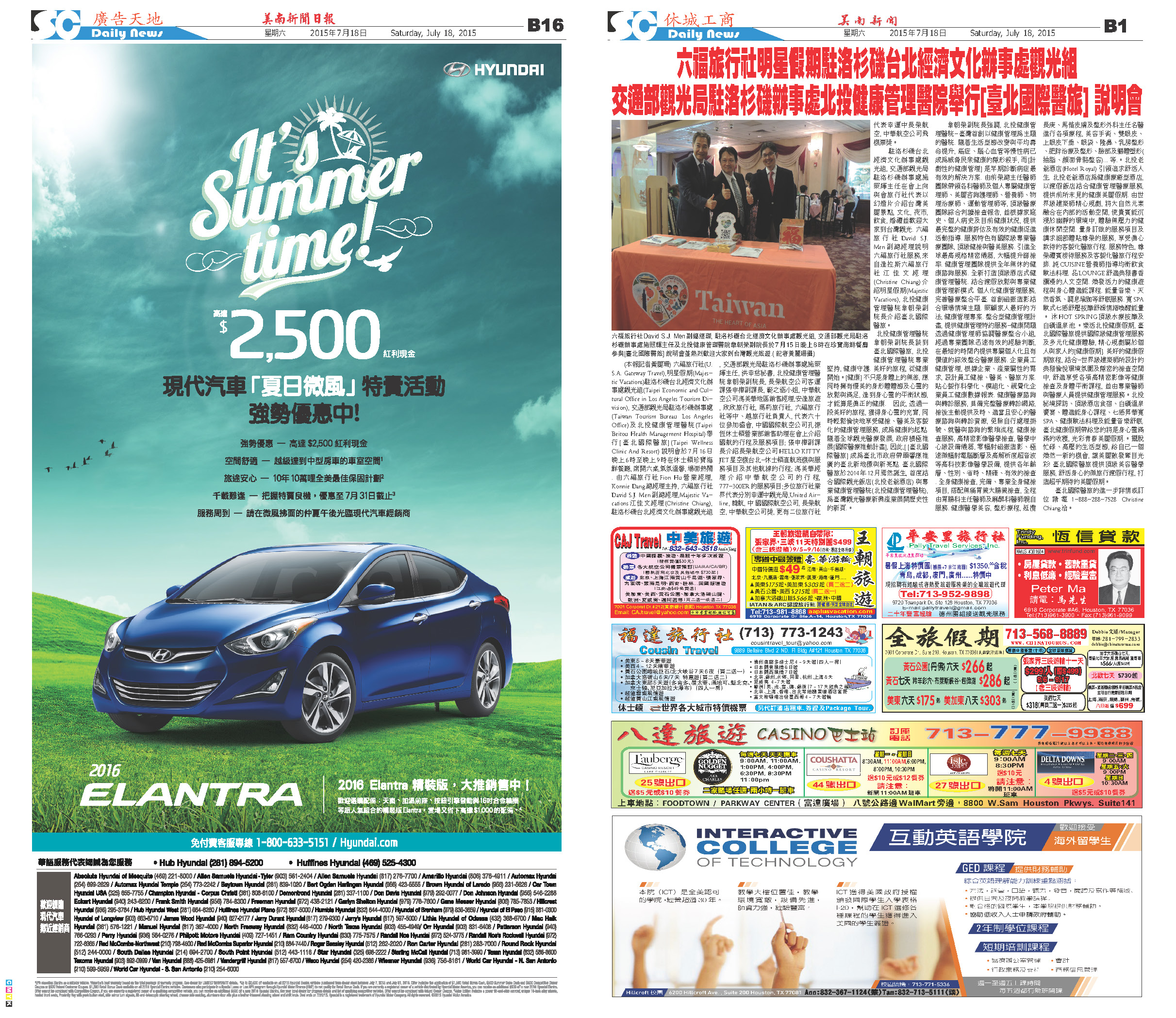C Section D Section150717 Epaper

 A Section B SectionC Section D Section150715 Epaper

 A Section B SectionC Section D Section150716 Epaper

 A Section B SectionC Section D Section150714 Epaper

 A Section B SectionC Section D Section